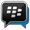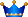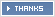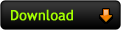﻿ 9iceunity » Forum | Waec Gce 2019 Physics Obj And Essay Answers - Nov/Dec Expo Runs

Welcome Guest, Please REGISTER or LOGIN To View Hidden Items

Hi, GuestAWOOF:- New MTN/ETISALAT/AIRTEL Unlimited Free Call/Browsing Code Now Available, Join Our BBM Channel Asap to Get it CLICK HERE (C0022EF41)JAMB 2019 KUNLESSI ANSWERS :-To Get Latest Answers About JAMB/WAEC/NECO/JUPEB 2019 ANSWERS, Kindly Click Here To join Us On WhatsApp Group (100% sure)
Forum » JAMB, WAEC, NECO PORTAL
Waec Gce 2019 Physics Obj And Essay Answers - Nov/Dec Expo Runs
Views: 378  |  Comments: 0 |  Posted: 07:19 Wed, 11 Sep 2019♦Kunlessi (¥ 17557 NU) Star:UltimateCreated Topics: 1722 Replies: 111
Posted on: 07:19 Wed, 11 Sep 2019+++++++++++++++++++++++++++++++++++
Exam Time:- Thursday, 12th September, 2019

Physics 2 (Essay) – 9:30 am – 11:00 am
Physics 1 (Objective) – 11:00am – 12:15pm
++++++++++++++++++++++++++++++++++

********************************
If You Get To Your Exam Hall Diz Morning Tell Them About Our Exam Runz Answer Via 9iceunity.com Always Invite Your Friends & Enemy To WWW.9ICEUNITY.COM
********************************

PHYSICS OBJ:-
1-10: BCCDBDCDDB
11-20: ABDBACABDA
31-40: DBCACACBCB
=•=•=•=•=•=•=•=•=•=•=

(2a)
H=ut+½gt²
Where H=80m, t=?
80=0(t) + ½(10)(t²)
80=0 + 5t²
80=5t²
80/5=t²
16=t²
t=√16
t=4s

(2b)
Range = U²/g
20²/10
=400/10
=40m

========================

(3)
F = KAV/L
FL = KAV
K = FL/AV
Where F = ma
= MLT-²
r = M, V = LT-¹
And A = M²

K = MLT-² × M/m² × LT-¹
K = LT-² ×L-¹T-¹
K = T-¹

====================================

6a) Ferromagnetism is the basic mechanism by which certain materials (such as iron) form permanent magnets, or are attracted to magnets.

6b)
i) Iron
ii) nickel
iii) cobalt

====================================
(8ai)
Pressure is defined as the force acting per unit area on a surface.

(8aii)
Density of liquid.
Depth of point from liquid surface.

(8aiii)
Total pressure = P + pgh
= 75.5cmHg + 1000×10×15
= 75.5cmHg + 150000
= 0.755mHg + 150000
= 150000.755

(8b)
In a tabular form between mass and weight

Under mass
(i) The same everywhere on the earth.
(ii) Scalar quantity.

Under weight
(i) Greater at the poles than at the equator ie it varies.
(ii) Vector quantity.

(8Ci)
(i) Both moving in opposite direction.
(ii) Both moving in the same direction but with one faster than the other.

(8cii)
Given Ma = 0.2kg; Mb = 0.5kg
Va = 5m/s, Vb = 3m/s
Using MaVa + MbVb = V(Ma+Mb)
0.2×(-5) + 0.5×3 = v(0.2 + 0.5)
-1 + 1.5 = 0.7v
0.7v = 0.5
V = 0.5/0.7
Common velocity = 0.71m/s

====================================

(11a)
An electric field is a region where an electric force is experienced by a charged body.

(11b)
In a tabular form
Under gravitational potential
(i) Scalar quantity
(ii) Unit is Joule per coulomb

Under gravitational field strength
(i) Vector quantity.
(ii) Unit is Newton per coulomb.

(11ci)
Equivalent capacitance = 5uf//(2uf + 2uf)
= 5uf // 4uf
= 5×4/5+4 uf
= 20/9 uf
= 2.22uf

(11cii)
Total energy stored = 1/2cv²
= 1/2×20/9×10^-6×12×12
=160×10^-6
= 0.00016Joules.

(11di)
Given: Im = 20×10^-3A; V = 25sin100πl
Capacitive reactance Xl = Vm/Im = 25/26×10^-3 = 1250ohms

(11dii)
1/2πfc = Xl
Where f = 50Hz
1/2×22/7×50c = 1250
314.286c × 1250 = 1
392857 c = 1
C = 1/392857 = 2.545×10^-6F
= 2.545uf

(11e)
A phasor diagram is a graphical way of representing the magnitude and directional relationship between two or more alternating quantities.

============================

(12a)
Thermoelectrons are electrons emitted at a high temperature such as one produced in a thermionic valve. They are negatively charged.

(12b)
(i) Low voltage battery
Used to heat the heater or heating element.
(ii) Target metal
Used to collect the emitted electrons.

(12Ci)
α - particles has a helium nuclei He while a β-particle has high energy electrons.

(12Cii)
α - particle has a charge of +2e while β-particle has a charge of -e.

(12di)
The radioactive decay law states that the probability per time that a nucleus will decay is constant, independent of time.

(12dii)
(i) Damage to skin.
(ii) Causes cancer.

(12ei)
Given 238 U --> m X + 2α
92 n
= 238 U --> m X + 2 4He
92 n 2
m+(2×4) = 238
m + 8 = 238
m = 238 - 8 = 230
Also
N + (2×2) = 92
N + 4 = 92
N = 92 - 4
n = 88

(12eiiYou Might Also Like This:-

Please dont forget to click the share button

### Replies

No replies yet! Be the first to reply and earn #3NU Instantly